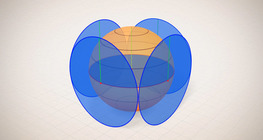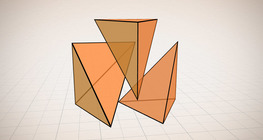Quantity: 0

Total: 0,00

# Volume of spheres (demonstration)

### Volume of spheres (demonstration)

The sum of the volume of the ´tetrahedrons´ gives an approximation of the volume of the sphere.

Mathematics

Keywords

volume of sphere, sphere surface, spherical segment, sphere, radius, tetrahedron, volume, mathematics

### Related items

Information Sphere#### Sphere

A sphere is the set of points which are all within the same distance from a given point in space.

Information Volume of spheres (Cavalieri´s principle)#### Volume of spheres (Cavalieri´s principle)

Calculating the volume of a sphere is possible using an appropriate cylinder and cone.

Information Perimeter, area, surface area and volume#### Perimeter, area, surface area and volume

This animation presents the formulas to calculate the perimeter and area of shapes as well as the surface area and volume of solids.

Information Ratio of volumes of similar solids#### Ratio of volumes of similar solids

This 3D scene explains the correlation between the ratio of similarity and the ratio of volume of geometric solids.

Information Surface area of spheres (demonstration)#### Surface area of spheres (demonstration)

The surface of a sphere consists of the set of points which are all at the same distance from a given point in space.

Information Solids of revolution#### Solids of revolution

Rotating a geometric shape around a line within its geometric plane as an axis results in a solid of revolution.

Information Volume and surface area (exercise)#### Volume and surface area (exercise)

An exercise about the volume and surface area of solids generated from a ´base cube´.

Information Volume of a tetrahedron#### Volume of a tetrahedron

To calculate the volume of a tetrahedron we start by calculating the volume of a prism.